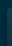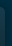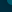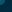# Beatles - Let It Be Chords & Tabs## Let It Be Chords & Tabs

Version: 10 Type: Chords
`Let It Be Chords`

```#-------------------------------PLEASE NOTE-------------------------------------#
# This file is the author's own work and represents their interpretation of the #
# song. You may only use this file for private study, scholarship, or research. #
#------------------------------------------------------------------#
Let it Be chords
The Beatles  *

C G Am F C G   F C Dm C  **

C              G                 Am          F
When I find myself in times of trouble, Mother Mary comes to me
C                 G              F C Dm C
Speaking words of wisdom, let it be

C             G                Am                F
And in my hour of darkness, she is standing right in front of me
C                 G              F C Dm C
Speaking words of wisdom, let it be

C      Am         G          F          C
Let it be, let it be, let it be, let it be
C                G              F C Dm C
Whisper words of wisdom, let it be

C               G               Am            F
And when the broken hearted people, living in the world agree
C                G              F C Dm C
There will be an answer, let it be

C                  G                Am                  F
But though they may be parted, there is still a chance that they may see
C                G              F C Dm C
There will be an answer, let it be
[ Tab from: https://www.guitartabs.cc/tabs/b/beatles/let_it_be_crd_ver_10.html ]
C      Am         G          F          C
Let it be, let it be, let it be, let it be
C                G              F C Dm C
There will be an answer, let it be
C      Am         G          F          C
Let it be, let it be, let it be, let it be
C                G              F C Dm C
Whisper words of wisdom, let it be

C      Am         G          F          C
Let it be, let it be, let it be, let it be
C                G              F C Dm C
Whisper words of wisdom, let it be

C                 G                Am                 F
And when the night is cloudy, there is still a light that shines on me
C                 G            F C Dm C
Shine on till tomorrow, let it be

C              G               Am          F
I wake up to the sound of music, Mother Mary comes to me
C                 G              F C Dm C
Speaking words of wisdom, let it be

C      Am         G          F          C
Let it be, let it be, let it be, let it be
C                G              F C Dm C
There will be an answer, let it be

C      Am         G          F          C
Let it be, let it be, let it be, let it be
C                G              F C Dm C  F Em Dm C
Whisper words of wisdom, let it be

* Alternate:

Capo III

C   = A
G   = E
Am  = F#m
F   = D
Dm  = Bm

** Alternate:

Capo I

C   = D
G   = A
Am  = Bm
F   = G
Dm  = Em

Set8
```